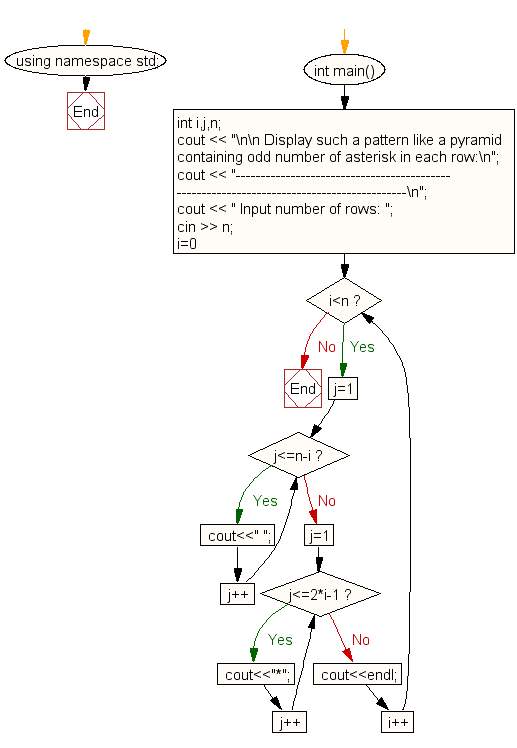﻿ C++ : Display the pattern like a pyramid using asterisk

# C++ Exercises: Display the pattern like a pyramid using asterisk and each row contain an odd number of asterisks

## C++: For Loop Exercise-42 with Solution

Write a C++ program that displays the pattern like a pyramid using asterisks, with odd numbers in each row.

Sample Solution:

C++ Code :

``````#include <iostream>
using namespace std;

int main()
{
int i,j,n;
cout << "\n\n Display such a pattern like a pyramid containing odd number of asterisk in each row:\n";
cout << "-----------------------------------------------------------------------------------------\n";
cout << " Input number of rows: ";
cin >> n;
for(i=0;i<n;i++)
{
for(j=1;j<=n-i;j++)
cout<<" ";
for(j=1;j<=2*i-1;j++)
cout<<"*";
cout<<endl;
}
}
``````

Sample Output:

``` Display such a pattern like a pyramid containing odd number of asterisk in each row:
-----------------------------------------------------------------------------------------
Input number of rows: 5

*
***
*****
*******
```

Flowchart:C++ Code Editor: